## Law of Conservation of Momentum:

The total momentum of an isolated system of particles is conserved. The law follows from the second and third law of motion.

Explain with an example: An important example of the application of the law of conservation of momentum is the collision of two bodies. Consider two bodies A and B, with initial momenta pA and pB. The bodies collide; get apart, with final momenta pA and pB respectively. By the Second Law(Where we have taken a common interval of time for both forces i.e. the time for which the two bodies are in contact.)
Since FAB = -FBA by the third law.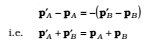which shows that the total final momentum of the isolated system equals its initial momentum. Notice that this is true whether the collision is elastic or inelastic. In elastic collisions, there is a second condition that the total initial kinetic energy of the system equals the total final kinetic energy.

### Linear Momentum:

It is defined as the quantity of motion contained in the body. Mathematically it is given by the product of mass and velocity. It is a vector quantity represented by p.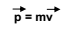### The principle of Conservation of Linear Momentum:

It states that in the absence of any external applied force total momentum of a system remains conserved.

Proof-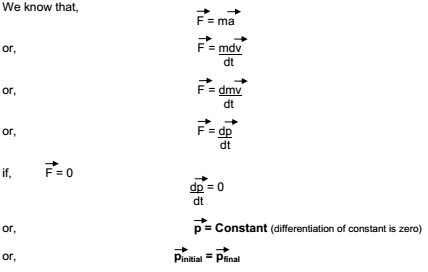Physical Application

1. The recoil of gun – when a bullet is fired in the forward direction gun recoils in the backward direction.
2. When a person jumps on the boat from the shore of the river, boat along with the person on it moves in the forward direction.
3. When a person on the boat jumps forward on the shore of river, boat starts moving in the backward direction.
4. In rocket propulsion fuel is ejected out in the downward direction due to which rocket is propelled up in vertically upward direction.

## Different Cases of Conservation of Linear Momentum

### Recoil of gun

Let mass of gun be mg and that of bullet be mb.
Initially both are at rest, hence their initial momentum is zero.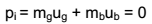Finally when bullet rushes out with velocity vg , gun recoils with velocity vb, hence their final momentum is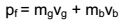Since there is no external applied force, from the principal of conservation of linear momentum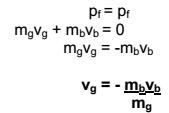From above expression it must be clear that
1. Gun recoils opposite to the direction of motion of bullet.
2. Greater is the mass of mullet mb or velocity of bullet vb greater is the recoil of the gun.
3. Greater is the mass of gun mg, smaller is the recoil of gun.

## Equilibrium of a particle:

Equilibrium of a particle in mechanics refers to the situation when the net external force on the particle is zero. According to the first law, this means that the particle is either at rest or in uniform motion.

If two forces F1 and F2, act on a particle, equilibrium requires

F1 = −F2

i.e. the two forces on the particle must be equal and opposite.

## Equilibrium of concurrent forces:

If the numbers of forces act at the same point, they are called concurrent forces. The condition or the given body to be in equilibrium under the number of forces acting on the body is that these forces should produce zero resultant.

The resultant of the concurrent forces acting on a body will be zero if they can be represented completely by the sides of a closed polygon taken in order.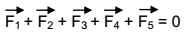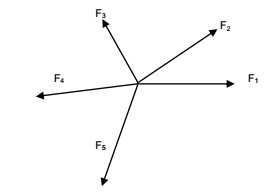Equilibrium of a body requires not only translational equilibrium (zero net external force) but also rotational
equilibrium (zero net external torque).